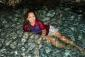# First Order Differential Equationfind the general solution

2y ln y dx - x (1 + 2x ln y) dy = 0

• Mathematics inside the configured delimiters is rendered by MathJax. The default math delimiters are $$...$$ and $...$ for displayed mathematics, and $...$ and $...$ for in-line mathematics.